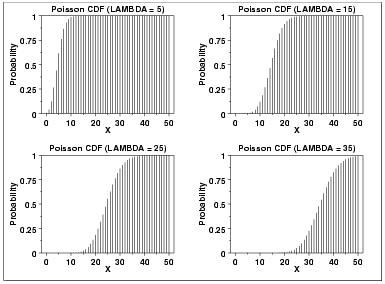# Statistics - Cumulative Poisson Distribution

${\lambda}$ is the shape parameter which indicates the average number of events in the given time interval. The following is the plot of the Poisson probability density function for four values of ${\lambda}$. Cumulative Distribution Function.## Formula

$${F(x,\lambda) = \sum_{k=0}^x \frac{e^{- \lambda} \lambda ^x}{k!}}$$

Where −

• ${e}$ = The base of the natural logarithm equal to 2.71828

• ${k}$ = The number of occurrences of an event; the probability of which is given by the function.

• ${k!}$ = The factorial of k

• ${\lambda}$ = A positive real number, equal to the expected number of occurrences during the given interval

### Example

Problem Statement:

A complex software system averages 7 errors per 5,000 lines of code. What is the probability of exactly 2 errors in 5,000 lines of randomly selected lines of code?

Solution:

The probability of exactly 2 errors in 5,000 lines of randomly selected lines of code is:

${ p(2,7) = \frac{e^{-7} 7^2}{2!} = 0.022}$